Practice the AP 9th Class Physical Science Bits with Answers Chapter 8 Gravitation on a regular basis so that you can attempt exams with utmost confidence.

## AP State Syllabus 9th Class Physical Science Bits 8th Lesson Gravitation with Answers

Question 1.
In a uniform circular motion ………………… is constant.
A) speed
B) force
C) gravitation
D) weight
A) speed

Question 2.
Which of the following quantity always changes in a uniform circular motion ?
A) direction of gravitation
B) direction of velocity
C) mass of the object
D) none
B) direction of velocityQuestion 3.
When a body is in uniform circular motion …………… force acts on it.
A) gravitational force
B) centrifugal force
C) centripetal force
D) normal force
C) centripetal force

Question 4.
Centripetal force acts
A) towards the centre of the circle
B) away from the centre of the circle
C) in a tangential direction
D) along the circular path
A) towards the centre of the circle

Question 5.
Acceleration due to gravity is independent of
A) speed of the object
B) gravitational force on the object
C) mass of the object
D) mass of the earth
C) mass of the object

Question 6.
Which of the following quantities remain constant in the planetory motion as seen from the sun
A) speed
B) angular speed
C) kinetic energy
D) angular momentum
D) angular momentum

Question 7.
A stone is released from an elevator going up with an acceleration a, the acceleration of the stone after release
A) a upwards
B) (g – A) upwards
C) (g – A) downwards
D) g downwards
D) g downwards

Question 8.
Relation between ‘g’ and ‘G’ is
A) g = G
B) g = GM/R2
C) G = gM/R2
D) GR2 = gM
B) g = GM/R2

Question 9.
The point where total weight appears to act is called
A) centre of gravity
B) centre of equilibrium
C) mid point
D) equilibrium point
A) centre of gravityQuestion 10.
If the line through the centre of gravity falls inside the base of the object, then the object will be
A) weightless
B) unstable
C) stable
D) freely falling
C) stable

Question 11.
If the line through the centre of gravity falls out side the base of the object, then the object will be
A) weightless
B) unstable
C) stable
D) freely falling
B) unstable

Question 12.
The motion of a body with constant speed in circular path is known as ……………
A) circular motion
B) rotatory motion
C) uniform circular motion
D) none of these
C) uniform circular motion

Question 13.
In uniform circular motion …………………….. is constant.
A) speed
B) velocity
C) acceleration
D) momentum
A) speed

Question 14.
The acceleration which can change only the direction of velocity of a body is called ………………..
A) retardation
B) deceleration
C) negative acceleration
D) centripetal acceleration
D) centripetal acceleration

Question 15.
The net force which can change only the direction of the velocity of a body is called ……………………
A) gravitational force
B) frictional force
C) centripetal force
D) electrical force
C) centripetal force

Question 16.
The direction of centripetal force is ……………….. the centre of the circle.
A) towards
B) away
C) left side of
D) right side of
A) towardsQuestion 17.
Motion of moon around the earth is …………………. motion.
A) vibrational
B) circular
C) rotational
D) uniform circular
D) uniform circular

Question 18.
The moon takes ………………….. for a complete revolution around the earth.
A) 25 days
B) 27.3 days
C) 30 days
D) 35 days
B) 27.3 days

Question 19.
Acceleration due to gravity is independent of …………………….. of the object.
A) mass
B) volume
C) weight
D) density
A) mass

Question 20.
The average position of weight distribution is simply the ……………………
A) centre of velocity
B) centre of momentum
C) centre of gravity
D) centre of acceleration
C) centre of gravity

Question 21.
The location of ……………………… is important for stability.
A) centre of velocity
B) centre of curvature
C) centre of momentum
D) centre of gravity
D) centre of gravity

Question 22.
Gravitational force can be called …………………….
A) contact force
B) non-contacting force
C) force at a distance
D) both B & C
D) both B & C

Question 23.
Two particles of same mass ‘m’ go round a circle of radius ‘r’ under the action of their mutual gravitational attraction. The speed of each particle is ……………………
A) $$\sqrt{\frac{\mathrm{GM}}{\mathrm{r}^{2}}}$$
B) $$\sqrt{\frac{\mathrm{GMm}}{\mathrm{r}}}$$
C) $$\sqrt{\frac{\mathrm{GM}}{4 \mathrm{r}}}$$
D) $$\sqrt{\frac{\mathrm{GM}^{2}}{\mathrm{r}}}$$
C) $$\sqrt{\frac{\mathrm{GM}}{4 \mathrm{r}}}$$Question 24.
Centripetal acceleration ……………..
A) $$\frac{\mathrm{v}}{\mathrm{r}}$$
B) $$\frac{\mathrm{v}^{2}}{\mathrm{r}}$$
C) $$\frac{\mathrm{v}^{2}}{\mathrm{r}^{2}}$$
D) $$\frac{\mathrm{v}}{\mathrm{r}^{3}}$$
B) $$\frac{\mathrm{v}^{2}}{\mathrm{r}}$$

Question 25.
Centripetal force ………………
A) $$\frac{\mathrm{mv}}{\mathrm{R}}$$
B) $$\frac{\mathrm{mv}^{2}}{\mathrm{R}^{3}}$$
C) $$\frac{\mathrm{mv}^{2}}{\mathrm{R}}$$
D) $$\frac{\mathrm{m}^{2} \mathrm{v}}{\mathrm{R}}$$
C) $$\frac{\mathrm{mv}^{2}}{\mathrm{R}}$$

Question 26.
Time period of a body moving around a body of radius R, with velocity v is …………………
A) T = $$\frac{2 \pi \mathrm{R}}{\mathrm{v}}$$
B) T = $$\frac{\mathrm{v}}{2 \pi \mathrm{R}}$$
C) T = $$\frac{2 \pi \mathrm{v}}{\mathrm{R}}$$
D) T= $$\frac{1}{2 \pi R v}$$
A) T = $$\frac{2 \pi \mathrm{R}}{\mathrm{v}}$$

Question 27.
Relation between force of attraction and distance between two objects is …………………
A) F ∝ $$\frac{1}{\mathrm{R}}$$
B) F ∝ $$\frac{1}{\mathrm{R}^{2}}$$
C) F ∝ $$\frac{1}{\mathrm{R}^{3}}$$
D) F ∝R
B) F ∝ $$\frac{1}{\mathrm{R}^{2}}$$

Question 28.
According to universal gravitational law, F =
A) $$\frac{\mathrm{GMm}}{\mathrm{R}^{2}}$$
B) $$\frac{\mathrm{GMm}^{2}}{\mathrm{R}^{2}}$$
C) $$\frac{\mathrm{GM}^{2} \mathrm{~m}}{\mathrm{R}^{2}}$$
D) $$\frac{\mathrm{GMm}}{\mathrm{R}^{3}}$$
A) $$\frac{\mathrm{GMm}}{\mathrm{R}^{2}}$$

Question 29.
Relation between time period of a satellite and radius of the planet ………………….
A) T2 ∝ R3
B) T ∝ R2
C) T2 ∝ R2
D) T3 ∝ R3
A) T2 ∝ R3

Question 30.
For a vertically projected body, a =
A) + g
B) g2
C) – g
D) None of these
C) – gQuestion 31.
Force F = ………………….
A) ma2
B) m2a
C) ma
D) m3a2
C) ma

Question 32.
For a freely falling body, a = ……………….
A) – g
B) + g2
C) + g3
D) + g
D) + g

Question 33.
Which of the following statement is true ?
Statement 1 : Velocity and acceleration of a body in a uniform circular motion are perpendicular to each other.
Statement 2 : The direction of the acceleration of a body in uniform circular motion is always towards its centre.
A) 1
B) 2
C) 1 and 2
D) None
C) 1 and 2

Question 34.
Assessment (A) : The net force which change only the direction of the velocity of a body is called centripetal force.
Reason (R) : Centripetal force exists only in uniform circular motion.
A) A and R are true
B) A and R are false
C) A is true but R is false
D) A is false but R is true
A) A and R are true

Question 35.
Which of the following is true ?
1) Earth attracts moon and it is gravitational.
2) The gravitational force acts as a centripetal force and makes the moon revolve around the earth in uniform circular motion.
A) 1
B) 2
C) 1 and 2
D) None
D) None

Question 36.
1) Force of gravitation acts between any two bodies in the universe.
2) Force of gravitation acts between objects nearer to earth and earth only.
3) Fgravity = G$$\frac{\mathbf{M}_{1} \mathbf{M}_{2}}{\mathbf{d}^{2}}$$
4) G = 6.67 x 10-11 Nm2 Kg-2
The wrong statement in the above is
A) 1
B) 2
C) 3
D) 2 and 4
B) 2

Question 37.
A body has constant speed while rotating in a circular path. Its direction of velocity
A) constant
B) changes
C) A or B
D) 0
B) changesQuestion 38.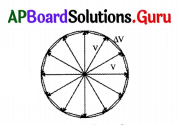If ΔV is decreases, then the path of the body is
A) in circular motion
B) in polygon motion
C) in spherical motion
D) none
A) in circular motion

Question 39.
Once while Sir Isaac Newton was sitting under a tree, an apple fell to the ground. Then some questions arose in his mind. Which questions would be those ?
a) Why did the apple fall to the ground ?
b) Does the apple eatable ?
c) What is the colour of the apple ?
d) What makes the apple to move ?
A) a
B) b and c
C) a and d
D) All
C) a and d

Question 40.
What will happen if the distance of a body from the centre of the earth increases ?
A) Force of gravity increases
B) Force of gravity decreases
C) Force of gravity does not change
D) None
B) Force of gravity decreases

Question 41.
If there is an attraction force between all objects why do a man not feel himself gravitating towards massive building ?
A) The attraction force between man and earth is greater than that of man and a building.
B) The attraction force between man and earth is less than that of man and a building.
C) The attraction force between man and earth is equal to attraction force between man and a building.
D) None
A) The attraction force between man and earth is greater than that of man and a building.

Question 42.
An apple falls because of the gravitational attraction of earth. The gravitational attraction of apple on the earth is …………………. to gravitational force of attraction of earth on the apple.
A) greater than
B) less than
C) equal
D) greater than or equal
C) equal

Question 43.
The direction of “g” is ………………….
A) towards sky
B) towards earth
C) horizontal to the earth
D) no direction
B) towards earth

Question 44.
If there is no gravity your weight becomes
B) zero
B) zeroQuestion 45.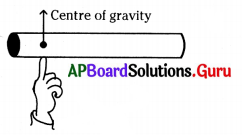What will happen to the scale ?
a) It will fall down.
b) It will not fall down.
A) a
B) b
C) a or b
D) we cannot say
B) b

Question 46.
When do you feel weightlessness ?
A) While climbing a tree.
B) While falling freely from a tree.
C) While walking on a road.
D) While running on a sand.
B) While falling freely from a tree.

Question 47.
If a paper and a book are dropped from same height at a time, they will reach the ground at same time
A) if their masses are equal
B) if there were no air resistance
C) if there is no gravitation
D) not possible
B) if there were no air resistance

Question 48.
The gravitational force of attraction between two bodies is F1. If the mass of each body is doubled and the distance between them is halved then the gravitational force between them is F2. Then
A) F1 = F2
B) F2 = 4F1
C) F2 = 8F1
D) F2 = 16F1
D) F2 = 16F1

Question 49.
Weight of a body on the surface of the earth is W. Then weight of the same body on the surface of a planet whose mass is double that of the earth and radius thrice that of the earth is
A) $$\frac{9 \mathrm{~W}}{2}$$
B) 18 W
C) $$\frac{2 \mathrm{~W}}{3}$$
D) $$\frac{2 \mathrm{~W}}{9}$$
D) $$\frac{2 \mathrm{~W}}{9}$$

Question 50.
Take an electric motor and fix a disc to the shaft of the electric motor. Place a small wooden block and switch on the motor. Wooden block speed is constant. The path of the wooden block is
A) linear
B) circular
C) uniform circular
D) zig zag
C) uniform circular

Question 51.
The apparatus to measure weight of a body is
A) simple balance
B) spring balance
C) electronic balance
D) above all
B) spring balanceQuestion 52.
The time period of a satellite (T) moving around the earth surface depends on
A) mass of the satellite
B) mass of the earth

Question 53.
Weight of a freely falling body is
A) equal to ‘g’
B) equal to ‘mg’
C) zero
D) equal to ‘mg + G’
C) zero

Question 54.
The acceleration produced due to gravitational force of the earth near the surface, is called ……………………
A) acceleration due to gravity
B) retardation
C) deceleration
D) acceleration due to object
A) acceleration due to gravity

Question 55.
The force due to gravity upon an object is known as its ………………
A) frictional force
B) gravitational force
C) weight force
D) both B & C
D) both B & C

Question 56.
The value of universal gravitational constant is found by ………………….
A) Henry Cavendish
B) Berzelius
D) Boyle
A) Henry Cavendish

Question 57.
Acceleration of moon towards earth is
A) 0.27 cm/s2
B)1.63 m/s2
C) 9.8 m/s2
D) 27.4 m/s2
A) 0.27 cm/s2

Question 58.
Acceleration of bodies on earth surface is
A) 981 m/s2
B) 981 cm/s2
C) 9.81 cm/s2
D) 0.98 cm/s2
B) 981 cm/s2Question 59.
A) 6.4 × 106 km
B) 6.4 × 106 m
C) 6.4 × 106 cm
D) 6.4 × 106 mm
B) 6.4 × 106 m

Question 60.
Time period of moon around the earth
A) 2.73 days
B) 27.3 days
C) 273 days
D) 2730 days
B) 27.3 days

Question 61.
Universal gravitational constant G =
A) 6.67 × 10-11 Nm2kg-2
B) 6.67 × 10-11 N2m2kg-2
C) 6.67 × 10-11 N2mkg-2
D) 6.67 × 10-11 Nm2kg2
A) 6.67 × 10-11 Nm2kg-2

Question 62.
Mass of earth is
A) 6 × 1022 kg
B) 6 × 1024 kg
C) 6 × 1020 kg
D) 6 × 1026 kg
B) 6 × 1024 kg

Question 63.
Distance of moon from the centre of earth is ………………….. cm.
A) 3.844 × 1010
B) 3.844 × 106
C) 3.844 × 105
D) 3.844 × 104
A) 3.844 × 1010

Question 64.
FC $$\frac{\mathbf{m v}^{2}}{\mathbf{R}}$$ is given. Here what is v ?
A) speed of a particle
B) weight of a particle
C) velocity of a particle
D) momentum of a particle
C) velocity of a particle

Question 65.

 set A set B v = u + at a s = ut + $$\frac{1}{2}$$ at2 h = $$\frac{\mathrm{gt}^{2}}{2}$$ v2 – u2 = 2as b

• Set A are the equations of uniform accelerated motion.
• Set B are the equations of a free fall body. Then a & b are

A) v = -gt, v2 = -2gh
B) v = gt, v2 = 2.gh
C) v = g – t, v2 – g2 = 2h
D) A or B
B) v = gt, v2 = 2.ghQuestion 66.
If 1 kg body weights 1.2 N and 2 kg body weights 2.4 N on a planet. Then the acceleration due to gravity on that planet is
A) 9.8 m/s2
B) 2 m/s2
C) 2.1 m/s
D) 1.2 m/s2
D) 1.2 m/s2

Question 67.
On observing the given figure, tell the theory explained by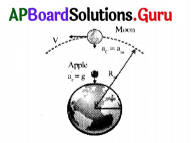A) Rutherford
B) Newton
C) Bohr
D) None
B) Newton

Question 68.
Name of the theory explained in the above figure.
A) Acceleration
B) Universal law of Gravitation
C) Direction of ‘g’
D) None
B) Universal law of Gravitation

Question 69.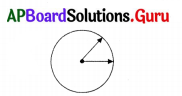Draw the change in velocity in this diagram.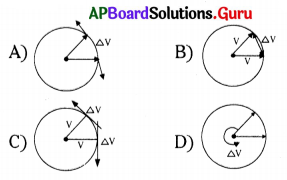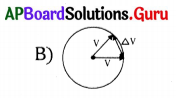Question 70.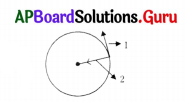A body in the uniform circular motion then the parts 1 and 2 represents
A) Time, Velocity
B) Speed, Velocity
C) Velocity, Acceleration
D) Acceleration, Velocity
C) Velocity, Acceleration

Question 71.Correct position of the centre of gravity located to the given object.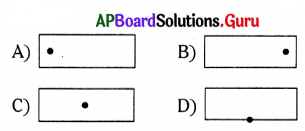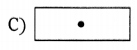Question 72.
Weight vector to the given diagram is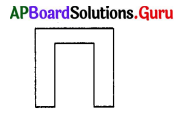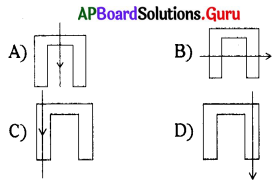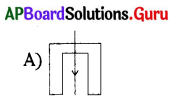Question 73.
The attraction force between two masses of 400 kg and 800 kg are with the distance of 400 meters apart is
A) G
B) 2G
C) G2
D) $$\frac{\mathrm{G}}{2}$$
B) 2GQuestion 74.
A body is projected vertically up. The distance covered in its last second of upward motion if g = 10 m/s2 is
A) 10 m
B) 2 m
C) 5 m
D) 0 m
C) 5 m

Question 75.
The time taken, by the body projected vertically up with a speed of 20 m/s to return back to the ground is (g = 10 m/s2)
A) 4 sec
B) 2 sec
C) 40 sec
D) 20 sec
A) 4 sec

Question 76.
Which of the following is easier to carry ?
A) Two buckets with same amount of water with both hands
B) One bucket with water with one hand
C) Both A or B
D) Two buckets with different amount of water with both hands
A) Two buckets with same amount of water with both hands

Question 77.
A person sitting in a satellite feels weightlessness because
A) the earth does not attract the objects in a satellite.
B) the normal force by the chair on the person balances the earth’s attraction.
C) the normal force is zero.
D) the person in satellite is not accelerated.
C) the normal force is zero.

Question 78.
From the top of a building 9 m high, water drops are falling at equal intervals of time, such that when the first drop reaches the ground, the fourth drop is just ready to fall. The distances between the successive drops in meters at that instance is
A) 1, 3, 5
B) 5, 3, 1
C) 1, 4, 9
D) 9, 4, 1
B) 5, 3, 1

Question 79.
Weight of 3 kg body is
A) 3 N
B) 3.2 N
C) 29.4 N
D) 19.6 N
C) 29.4 N

Question 80.
Gravitational force between two bodies exists
A) when they are in contact only
B) when they are not in contact only
C) any of the above two cases
D) none of these
C) any of the above two casesQuestion 81.
When a stone moves up, its speed ……………………..
A) increases
B) decreases
C) does not change
D) none of these
B) decreases

Question 82.
When a body is freely suspended, the weight is evidenced by ………………………
A) gravitational force
B) normal force
C) tension
D) none of these
C) tension

Question 83.
When a body falls freely then it experiences …………………….
A) weight
B) mass
C) weightlessness
D) none of these
C) weightlessness

Question 84.
Gravitational force between any two bodies is always ………………… type only.
A) attractive
B) repulsive
C) both
D) none of these
A) attractive

Question 85.
The time period of a satellite depends on the …………………. of the planet.
A) diameter
C) mass of satellite
D) both A & B
D) both A & B

Question 86.
In uniform circular motion, which of the following is/are correct
i) Net force can change only the direction of the velocity of the body
ii) Net force is always directed towards the centre
iii) Net force is called centripetal force
A) i, ii
B) ii, iii
C) i, iii
D) i, ii, iii
D) i, ii, iii

Question 87.
The direction of acceleration in uniform circular motion is
A) Tangential
B) Towards centre
C) Away from centre
D) No direction
B) Towards centreQuestion 88.
When we move away from the surface of the earth, the acceleration due to gravity value
A) Decreases
B)Increases
C) Remains the same
D) Zero
A) Decreases

Question 89.
Assertion (A) : weight of an object is less on moon then earth
Reason (R): earth is heavier than moon
A) Both A and R are correct, R is the correct explanation of A
B) Both A and R are correct, R is not the correct explanation of A
C) A is correct, R is not correct
D) A is not correct, R is correct
B) Both A and R are correct, R is not the correct explanation of A

Question 90.
Correct order of following options to make a meaningful experiment is
P) Suspend the object from one point, draw vertical line
Q) Centre of gravity lies where two lines intersect
R) Take a India map made of steel
S) Suspend the object from some other point, draw vertical line
A) P, Q, R, S
B) R, S, P, Q
C) R, P, S, Q
D) Q, R, P, S
C) R, P, S, Q

Question 91.
What is initial velocity of freely falling body ?
A) 9.8 m/s
B) 8.9 m/s
C) 0 m/s
D) 10 m/s
C) 0 m/s

Question 92.
What is the direction of acceleration due to gravity ?
A) Always downwards
B) Always upwards
C) Some times downwards and some times upwards
D) In the direction of object motion
A) Always downwards

Question 93.
Assertion : 10kg body weight 98N Reason : weight W – m\$
A) Both A and R are correct and R support A
B) Both A and R are correct and R does not support A
C) A correct, R incorrect
D) A in correct, R – correct